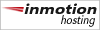#### Luonnollinen logaritmi  ln(2)

Ei kovin vanha, sillä sen käsitteen ottivat käyttöön Saint-Vincent ja de Sarasa ennen vuotta 1649!

ln(2) = 0.69314718055994530941723212145817656807550013436025...

The alternating sum   1 - 1/2 + 1/3 - 1/4 + 1/5 - 1/6 + 1/7 - 1/8+ ...
The first few decimals of this pervasive constant are worth memorizing!

...by shortening the labors, doubled the life of the astronomer.
- Pierre Simon de Laplace (1749-1827) about logarithms.

You have no idea, how much poetry there is in the calculation of a table of logarithms!
- Karl Friedrich Gauss (1777-1855), to his students.

 The two following programs are short C program from Pascal Sebah (Jan 2000) to compute 2400 decimal places of log(2). In the two algorithms, the number of digits is given by log10(a).n where a is the working base (here a = 103 or less if you want to compute more digits) and c is the numbers of iteration required, the size of the array f[] is c+1. The first program is based on the hypergeometric representation of x-1log(1-x) with x = 1/2 (see ) : ``` main(){ int a=1000,b=0,c=7973,d,f,n=800,k; for(;b

Powered Byand CodeIgniter 3.1.11